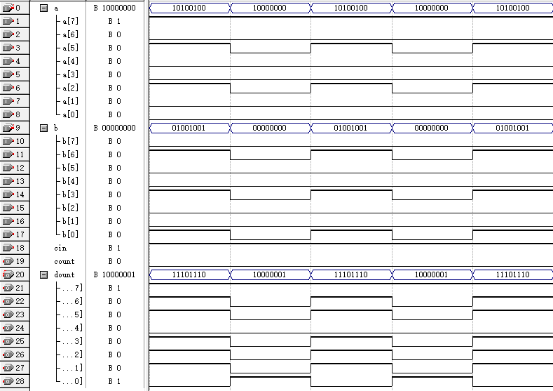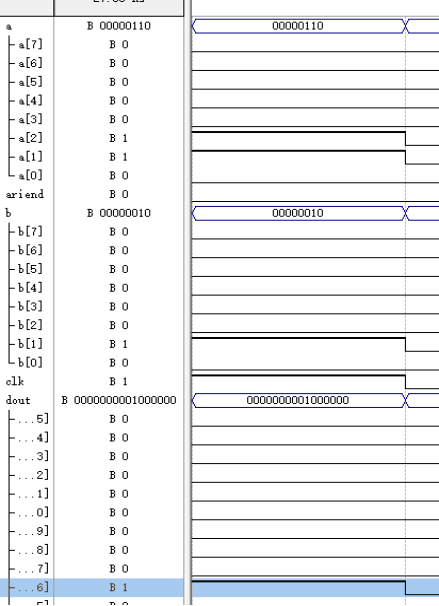• 二进制为什么是八位
千次阅读
2019-02-21 16:49:02

八位（8bit）是一个字节，计算机中只要一个字节就可以存放ASCII编码，就是所有的数字、大小写字母和一些特殊字符（总共255个）。所以在计算机中对用户来说有意义的单位就是字节，这是我们可以看懂的。
八位的二进制数最大值就是255，刚好表示255个ASCII字符。
我们汉字使用的是unicode编码的，unicode编码要用两个字节，所以要存放16位二进制数才能代表一个unicode字符。
另外还有UTF8编码占四个字节，等等。

更多相关内容
• 八位二进制转三位十进制电路multisim源文件，十进制数字用数码管显示，multisim13及以上版本的软件可以正常打开仿真。二进制转十进制
• 进行两个四位二进制数的运算。 b.算术运算： A+B,A-B,A+1,A-1。 c.逻辑运算： A and B,A or B,A not, A xor B。 2、4 位输入 A3—A0、B3---B0 用开关设置输入。 3、8种算术逻辑运算通过 3 位功能选择开关选择某一种...
• 初学者，文件过程：先设计一位的加法计数，再一个个进位达成八位二进制加法，若要改成十进制的只需要修改加法部分语言将二进制改成十进制即可
• 用移位相加的方法设计一个8位二进制串行乘法器。基于EP4CE1022C8芯片，于Quartus Ⅱ 13.1中实现。包含仿真文件，上板子验证成功。此西电EDA课设大作业，实验报告见博客，仅供参考。
• 八位二进制与十进制、十六进制对照表.doc，方便项目中排查使用，下载后对比查看。 本人亲自整理验证，查看无误可用。供大家下载使用ascii
• 要求输入一个小于256的十进制整数，要将其转换一个必须有八位数字的二进制
• 没有任何标准限制者二进制只能有八位，最开始为了保存26个英文字母以及一些可见符号和一些控制字符，老美的专家给出了一套7位一字节的编码方式，就是著名的ASCII编码。这套编码只能容纳英文字符，后来为了现实需要...

请改一下问题：电脑存储数据为什么以8位为一个存储单位？

没有任何标准限制者二进制只能有八位，最开始为了保存26个英文字母以及一些可见符号和一些控制字符，老美的专家给出了一套7位一字节的编码方式，就是著名的ASCII编码。这套编码只能容纳英文字符，后来为了现实需要只好加了一位，变成如今广泛使用的8位编码。

由于字符采用8位表示，编程自然以对应的长度来读取和计算最方便高效，因为不需要进行额外的转换。

但8位(一字节)表示的范围是0-255共256个数字，多数情景无法胜任，所以有了2字节的short，4字节的int以及8字节的long。注意int并不一定是4字节，int被定义为“机器自然长度”，所谓机器自然长度是指与机器处理数据时采用的位长相同，16位机器就是16位即2字节，32位机器就是4字节，64位机器就是8字节，而机器位长是指机器的总线宽度，总线宽度决定了机器一个时钟周期内可处理的数据量，总线一般分为数据总线、地址总线及控制总线，前二者一般位长是一样的，且如果笼统说总线位宽时一般只指前二者。所以程序员要定义一个数字首先想到的一定是int，这样可以保证自己的操作可以在一个时钟周期里完成，又不浪费总线的带宽。

而题主所说的2进制被限制为8位显然是无法成立的，只有字节被定义为8位。8位一个单元多用来存储ASCII字符，但为了兼容多国语言现在很多操作系统内码多用UICODE字符，只用8位存储一个基本数据的情况越来越少，8位一字节现在更多的只是用来作为表示存储容量的单位而已。

手机码的字，网页有问题，难受啊。

展开全文java位宽
• 设计、实现八位二进制数全加器 设计思路 总体设计三输入，两输出。具体：实体声明部分描述电路模块的端口，即指定输入输出口及其大小。设计具有8位位宽的矢量或总线端口信号a，b以及标准一位输入的cin。然后在...

实验题目
设计、实现八位二进制数全加器

设计思路
总体设计为三输入，两输出。具体：实体声明部分描述电路模块的端口，即指定输入输出口及其大小。设计具有8位位宽的矢量或总线端口信号a，b以及标准一位输入的cin。然后在结构体描述部分对电路模块的功能进行描述，指明整个电路时如何运作的：对3个二进制的9位矢量数进行加法运算输出到data。
使用两个四位二进制加法器组成。

功能设计
实现八位二进制数加法，并保存可能存在的溢出值。

波形图源代码

library ieee;
use ieee.std_logic_1164.all;
use ieee.std_logic_unsigned.all;
port (a,b : in std_logic_vector(7 downto 0);
count : out std_logic;
cin : in std_logic;
dount : out std_logic_vector(7 downto 0) );
architecture bhv of add8 is
signal data :std_logic_vector(8 downto 0);
begin
data <= ('0' & a) + ('0' & b) + ("00000000" & cin);
count <= data(8);
dount <= data(7 downto 0);
end architecture bhv;


实验题目
设计、实现八位无符号二进制数乘法器

设计思路
八位乘法器由八位全加器以时序逻辑构成，通过逐项移位相加原理实现乘法。乘数的各位数码从被乘数的最低位开始依次相乘，每相乘一次得到部分积，若为此位的数码为1，则乘数左移后与上一次的和相加；若为0，左移后以全零相加，直至被乘数的最高位，所有的部分积被加过一次可以得到相应的乘积。由于乘数的每一位不是0就是1，对应的部分积不是0就是和被乘数相等的部分积，所以实际作部分积相加这一步时，只要根据乘数的对应位判断，如过该位为1，则将累加器中的数据加上被乘数再移位；如该位为0时，就不加被乘数而直接移1位。然后对乘法器的进行自顶而下设计，把乘法器的设计分成几个功能模块，进而逐步细化。该任务需了解各个模块的引脚含义以及内部构造，并对各个模块的实现功能进行分析，通过VHDL对这些模块进行编程，调试。最后在QuartusⅡ进行模拟仿真，得出正确的运行结果。

功能设计
本设计采用移位和加法来实现两个8位二进制数相乘，使用VHDL语言完成八位乘法器，以及如何做二进制位相乘的运算过程。该乘法器是由八位加法器构成八位乘法器，通过逐项移位相加来实现乘法功能，并以QuartusⅡ软件工具进行模拟。

波形图源代码：

1．library ieee;                               //四位二进制并行加法器
use ieee.std_logic_1164.all;
use ieee.std_logic_unsigned.all;
port( cin:in std_logic;
a,b:in std_logic_vector(3 downto 0);
s:out std_logic_vector(3 downto 0);
cout:out std_logic);
end;
architecture one of add4 is
signal sint,aa,bb:std_logic_vector(4 downto 0);
begin
aa<='0' & a;
bb<='0' & b;
sint<=aa+bb+cin;
s<=sint(3 downto 0);
cout<=sint(4);
end;

2．library ieee;          //由两个加法器结合成八位加法器;
use ieee.std_logic_1164.all;
use ieee.std_logic_unsigned.all;
port( cin:in std_logic;
a,b:in std_logic_vector(7 downto 0);
s:out std_logic_vector(7 downto 0);
cout:out std_logic);
end;
architecture one of add8 is
port( cin:in std_logic;
a,b:in std_logic_vector(3 downto 0);
s:out std_logic_vector(3 downto 0);
cout:out std_logic);
end component;
signal carryout: std_logic;
begin
u1:add4 port map(cin,a(3 downto 0),b(3 downto 0),s(3 downto 0),carryout);
u2:add4 port map(carryout,a(7 downto 4),b(7 downto 4),s(7 downto 4),cout);
end;

3.library ieee;                  //一位乘法器;
use ieee.std_logic_1164.all;
use ieee.std_logic_unsigned.all;
entity andarith is
port( abin:in std_logic;
din:in std_logic_vector(7 downto 0);
dout:out std_logic_vector(7 downto 0));
end;
architecture one of andarith is
begin
process(abin,din)
begin
for i in 0 to 7 loop
dout(i)<=din(i) and abin;
end loop;
end process;
end;

4.library ieee;          //乘法运算控制器
use ieee.std_logic_1164.all;
use ieee.std_logic_unsigned.all;
entity arictl is
port( clk,start:in std_logic;
clkout,rstall,ariend:out std_logic);
end;
architecture one of arictl is
signal cnt4b:std_logic_vector(3 downto 0);
begin
rstall<=start;
process(clk,start)
begin
if start='1' then cnt4b<="0000";
elsif clk'event and clk='1' then
if cnt4b<8 then        //小于8：计数，等于8：表明乘法运算结束
cnt4b<=cnt4b+1;
end if;
end if;
end process;
process(clk,cnt4b,start)
begin
if start='0' then
if cnt4b<8  then
clkout<=clk;    ariend<='0';
else clkout<='0'; ariend<='1';
end if;
else clkout<=clk; ariend<='0';
end if;
end process;
end;

5.library ieee;
use ieee.std_logic_1164.all;
use ieee.std_logic_unsigned.all;
entity reg16b is
port( clk,clr:in std_logic;
d:in std_logic_vector(8 downto 0);
q:out std_logic_vector(15 downto 0));
end;
architecture one of reg16b is
signal r16s:std_logic_vector(15 downto 0);
begin
process(clk,clr)
begin
if clr='1' then r16s<="0000000000000000";
elsif clk'event and clk='1'  then
r16s(6 downto 0)<=r16s(7 downto 1);
r16s(15 downto 7)<=d;
end if;
end process;
q<=r16s;
end;

6.library ieee;                         //8位右移寄存器：在时钟信号作用下，进行输入值的移位与锁存。
use ieee.std_logic_1164.all;
use ieee.std_logic_unsigned.all;
entity sreg8b is
din:in std_logic_vector(7 downto 0);
qb:out std_logic);
end;
architecture one of sreg8b is
signal reg8:std_logic_vector(7 downto 0);
begin
begin
if clk'event and clk='1'  then
if load='1' then reg8<=din;
else reg8(6 downto 0)<=reg8(7 downto 1);
end if;
end if;
end process;
qb<=reg8(0);
end;

7.library ieee;               //8位乘法器顶层设计
use ieee.std_logic_1164.all;
use ieee.std_logic_unsigned.all;
entity mult8x8 is
port( clk:in std_logic;
start:in std_logic;
a,b:in std_logic_vector(7 downto 0);
dout:out std_logic_vector(15 downto 0);
ariend:out std_logic);
end;
architecture struc of mult8x8 is
port( cin:in std_logic;
a,b:in std_logic_vector(7 downto 0);
s:out std_logic_vector(7 downto 0);
cout:out std_logic);
end component;
component andarith is
port( abin:in std_logic;
din:in std_logic_vector(7 downto 0);
dout:out std_logic_vector(7 downto 0));
end component;
component arictl is
port( clk,start:in std_logic;
clkout,rstall,ariend:out std_logic);
end component;
component reg16b is
port( clk,clr:in std_logic;
d:in std_logic_vector(8 downto 0);
q:out std_logic_vector(15 downto 0));
end component;
component sreg8b is
din:in std_logic_vector(7 downto 0);
qb:out std_logic);
end component;
signal gndint  :std_logic;
signal intclk  :std_logic;
signal rstall  :std_logic;
signal qb  :std_logic;
signal andsd  :std_logic_vector(7 downto 0);
signal  dtbin :std_logic_vector(8 downto 0);
signal  dtbout :std_logic_vector(15 downto 0);
begin
dout<=dtbout; gndint<='0';
u1:arictl port map( clk,start,intclk,rstall,ariend);
u2:sreg8b port map(intclk,rstall,b,qb);
u3:andarith port map(qb,a,andsd);
u4:add8 port map(gndint,dtbout(15 downto 8),andsd,dtbin(7 downto 0),dtbin(8));
u5:reg16b port map(intclk,rstall,dtbin,dtbout);
end;


学习心得
通过本次课外实验项目，我了解了如何使用QuartusⅡ软件、加深了对VHDL语言结构的认识以及如何设计加法乘法器。虽然途中出现了一些困难与问题，比如说运行波形图时结果出现毛刺，设置为功能仿真后又出现Error等等的问题。但是我还是在查询资料的过程中，通过对同一个工程下不同程序的连接，逻辑结构的梳理，对最后结果的测试验证等方面的学习，加深了对整个代码及软件的认识。。此外，在团队中，我们独立思考，互相分享经验和进步，这也改善了自己的各项能力。

展开全文• //将一个八位二进制数转换十进制 #include<iostream> #include<cstdio> using namespace std; int convert(char *a){ int sum=0; int q=1; for(int i=7;i>=0;i--){ if(a[i]=='1'){ sum+=q; ...

代码：

//将一个八位的二进制数转换为十进制

#include<iostream>
#include<cstdio>

using namespace std;

int convert(char *a){
int sum=0;
int q=1;
for(int i=7;i>=0;i--){
if(a[i]=='1'){
sum+=q;
}
q*=2;
}

return sum;
}

int main(){
char a;
for(int i=0;i<8;i++){
scanf("%c",&a[i]);
}

return 0;
}


展开全文二进制
• 八位二进制就是8个按顺序排列的二进制数。例如：11111000，00000001，00000101等。进制(binary)在数学和数字电路中指以2基数的记数系统，以2基数代表系统是二进位制的。这一系统中，通常用两个不同的符号0(代表...
• 二进制转化十进制数 用字符型接收二进制的0、1然后判断是否‘1’ 功能实现 代码片. #include<iostream> using namespace std; double powe(double x, int n); int main() { int su = 0; char a; for ...
• //十进制转二进制 cout << "输入一个十进制整数" << endl; cin >> n; for (i = 0;i < 8;i++) { a[i] = n % 2; n = n / 2; } for (i=7;i>=0;i--) cout <<.
• //根据Value中的十进制数，返回一个存有二进制每一位的数组 function ten_two(c_Value){ 　var two=parseInt(c_Value).toString(2); 　var Point=[]; 　if(two.length<8){ 　for(var j=0;j<(8-two.length...
• 八位二进制数转化十进制数输出 分析：将二进制转化十进制，只要将二进制数的每一位乘以该位的权然后相加 */ #include "iostream" using namespace std; //计算x的n次方 double power(double x,int n); int...
•   八位二进制可表示的范围：     原码：-127~127     反码：-127~127     补码：-128~127 原因 原码   第一位是符号位（负数1，正数0）。   也就是 1111 1111b 中首位1表示这个数是负数。 ...数据结构
• 功能： Multisim八位二进制转三位十进制 说明： 用于练习、参考、学习使用，提高数电设计技术 运行版本：multisim14向下兼容
• ## 八位二进制乘法器VHDL

千次阅读 多人点赞 2020-11-02 21:56:08
八位二进制乘法器VHDL工程文件一、 实验目的二、设计要求三、实验仪器与环境四、实现原理五、系统设计及仿真六、顶层设计：七、结果仿真：附录： 工程文件 点击下载八位二进制乘法器工程文件 一、 实验目的 熟习...
• 将一个数字字符串变为八位二进制数然后相加，最后结果一个二进制数相加结果 //测试字符串 String xxx = "1234"; //将字符串转换字符数组 char[] arr = xxx.toCharArray(); //得到数组长度，避免多次调用 ...java 字符串
• 组成原理课程设计层次化设计方法二进制计数器八位加法器.docx组成原理课程设计层次化设计方法二进制计数器八位加法器.docx组成原理课程设计层次化设计方法二进制计数器八位加法器.docx组成原理课程设计层次化设计...
• 组成原理课程设计层次化设计方法二进制计数器八位加法器.pdf组成原理课程设计层次化设计方法二进制计数器八位加法器.pdf组成原理课程设计层次化设计方法二进制计数器八位加法器.pdf组成原理课程设计层次化设计方法...
• 74185是用途很广的芯片，它可以将二进制数转换成十进制BCD码。 但是，无论Multism还是Proteus，均没有74185的仿真模型。作者参考74185的Datasheet，利用分立元件制作了74185仿真电路，可将8位二进制数转换十进制BCD...
• 整数转化8位二进制详解（包括正数和负数） 适用范围-127~+127java
• 一、4位二进制和4位二进制补码对照表.png 二、8位二进制和8位二进制补码对照表.jpg
• 为什么八位二进制数表示范围为-128~+127？ 在昨天的文章中我们讲解了什么原码反码补码，以及计算机中为什么要使用补码，在文章最后的时候我们说了一个问题，八位二进制（在Java中就是byte类型）的取值范围是从-128到...二进制
• 例如一个十进制数64580想转化十六进制并赋定时器的低八位和高八位给TL0,TH0 那么高八位TH0就等于64580/256 低八位TL0就等于64580%256 在这里我用一个类比的方法去想的，比如一个十六进制数0001 1001即0x19想...
• 读取csv文件中的十进制常数（有正有负），将其转换成8位二进制数存入txt文件中，在单片机引脚输出中可能会用到。 import numpy as np import pandas as pd import os import types import csv from shutil import ...python
• 2019独角兽企业重金招聘Python工程师标准>>> ...
•大数据...